Next: Si-doped system Up: 6.2 Spin-Peierls material CuGeO Previous: Nominally pure CuGeO

### Zn-doped systems

In Fig.56, zero-field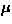SR spectra of the Zn-doped compounds are shown. Contrary to the pure system, we observed Gaussian-like muon spin relaxation, suggesting that static local fields are induced by Zn doping. In the ordered phase (), we confirmed that the spectra converges to the 1/3 of the full amplitude; the existence of the `1/3-component' is an unambiguous signature of static freezing of moments, as has been explained in Chapter 3.In order to estimate the characteristic field distribution width (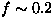), we phenomenologically analyzed the spectra with a stretched exponential function,. Since the stretching power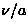represents the shape of the field distribution, we assumed that it is temperature independent; we obtainedfrom the lowest temperature data and fixed it all through the fit (and 2.00 for the x=2, 4 and 8 % sample, respectively.) Near the Néel temperature, the relaxation amplitude (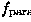ordered volume fraction) decreased as the temperature go through the transition temperature; this result suggests a distribution of. We introduced a paramagnetic volume fraction (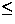), and analyzed the spectra with the functional form of:

where the first term represents the muons which land in the paramagnetic volume fraction and the second term, in the ordered fraction. The fits are shown in Fig.56 as the solid lines.

In Fig.57, we show the field width (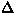) and the paramagnetic volume fraction () as a function of temperature. The field width () saturates at low temperatures, being consistent with the static order. In the inset of Fig.57a, the saturation magnitude of the field width [] are plotted as a function of the Zn concentration (x). It was found thatmimics the Zn concentration (x) dependence of(Fig.53).

The temperature dependence of the paramagnetic fraction ()suggests a distribution of Néel temperatures; with an assumption of Gaussian distribution to(), the average Néel temperatureand the spread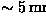have been deduced as shown in Fig.57b.In Fig.58, results of longitudinal field (LF) decoupling measurements are shown. The spectra exhibited the characteristics of static relaxation, namely, time independent muon spin polarization atand the decoupling field comparable to the field distribution width (). The solid lines in Fig.58 are static Gaussian Kubo-Toyabe function (eq.19), using the field-width parameter () obtained from the stretched exponential fit shown in Fig.56b. In small LF's, the Gaussian Kubo-Toyabe function described the recovery at long times relatively well; the deviation at higher LF's may suggest that there exists a larger local field component than true Gaussian distribution assumes.Next: Si-doped system Up: 6.2 Spin-Peierls material CuGeO Previous: Nominally pure CuGeO